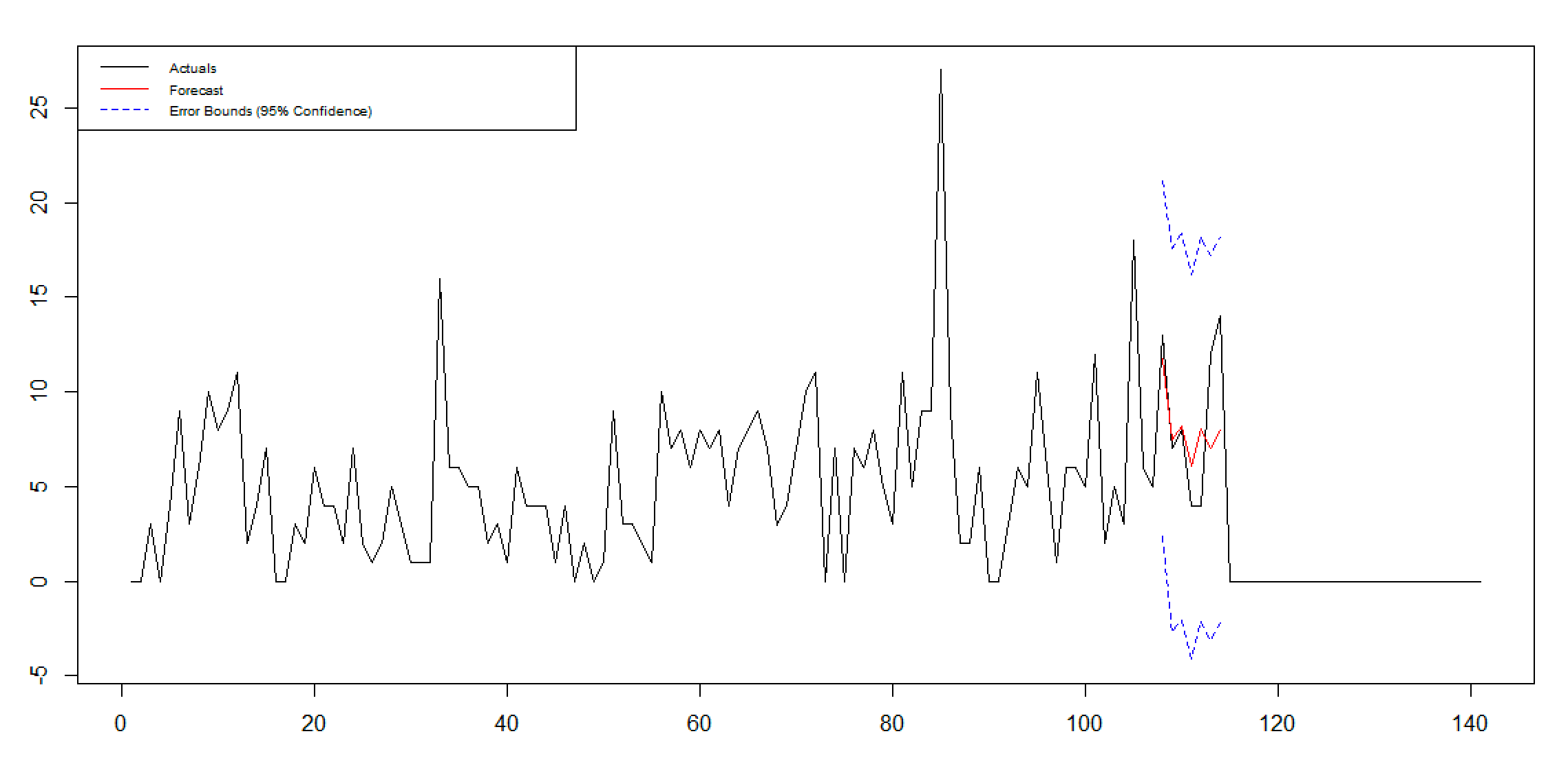# #StackBounty: #r #time-series #arima auto.arima returns a non-seasonal model even though I am forcing seasonality

### Bounty: 100

Per this post, you can force seasonality in auto.arima by selecting D=1.

I have a weekly time series which looks like it might (or might not) have a seasonal component (I have a priori reasons for thinking it might have a seasonal component).

``````Data <- as.ts(Data\$Sales,order.by=Data\$Date, frequency=52)
Train <- window(Data,start=3,end=107)
Test <- window(Data,start=108,end=116)
``````

I tried manually fitting a seasonal model:

``````fit <- arima(Train, order=c(2,0,1) , seasonal = list (order= c(0,1,0) , period = 52))
``````

gave an “OK” forecast (see first graph).So I tried improving on it by using auto.arima to find the best model.

``````AutoFit <- auto.arima(Train)
``````

This returned an ARIMA(1,1,1) model, which I then fit using:

``````#fit <- arima(Train, order=c(1,1,1))
``````

But this gave worse results than the seasonal model I selected manually (see second graph).So I tried to force seasonality by running:

``````AutoFit <- auto.arima(Train, D=1)
``````

But I still get the same ARIMA(1,1,1) model.

Why is auto.arima not trying to fit a seasonal model, even why I try to force it?

I’ve also tried:

``````AutoFit <- auto.arima(Train, seasonal=TRUE, D=1)
``````

and

``````AutoFit <- auto.arima(Train, seasonal=TRUE, start.P=0, start.Q=0 , D=1)
``````

Get this bounty!!!

This site uses Akismet to reduce spam. Learn how your comment data is processed.